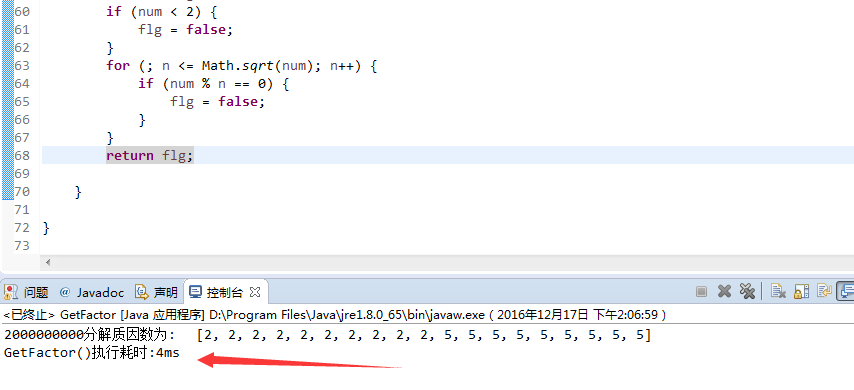# java分解质因数的方法 [源代码分享]

```import java.util.ArrayList;

/**
* 分解质因数
* @url https://www.yangshengliang.com/biancheng-kaifa/java-jiancheng/527.html
* @author fedkey
* @blog www.yangshengliang.com
*
*/

public class GetFactor {
private static ArrayList<Integer> factorArr = new ArrayList<>(); // 存放质因数

public static void main(String[] args) {
long startTime = System.currentTimeMillis();
int num = 2000000000;
GetFactor.getFactor(num);
// 计时
long endTime = System.currentTimeMillis();
System.out.print(num + "分解质因数为:\t" + factorArr);
System.out.print("\n");
System.out.println("GetFactor()执行耗时:" + (endTime - startTime) + "ms");

}

public static void getFactor(int num) {

if (isPrime(num)) {
}
for (int n = 2; n <= num / 2; n++) {
if (isPrime(n) && num % n == 0) {
int c = num / n;// 商
if (!isPrime(c)) {
getFactor(c);

} else {

}
break;

}

}
}

/**
* 判断是否是质数
*
* @param num
* @return
*/
public static boolean isPrime(int num) {
int n = 2;
boolean flg = true;
if (num < 2) {
flg = false;
}
for (; n <= Math.sqrt(num); n++) {
if (num % n == 0) {
flg = false;
}
}
return flg;

}

}```

1.用>=2的质数做除(相对于合数,质数毕竟是少数,避免不必要的计算)数去除,如果能除完，则该质数是该合数的一个质因数,加入到 factorArr中

2.检测商是否是质数，如果是质数，将直接放入结果集 factorArr中,程序结束;

3.如果商是合数，则再次分解(递归调用getFactor(int num)方法)，直到商为质数不可再分为止。2条评论
• 衣皇后

2016年12月25日 下午2:33

掐指一算，这个博客能风光一百年！

• 三五营销

2016年12月21日 下午1:52

偶然来访，受益良多！